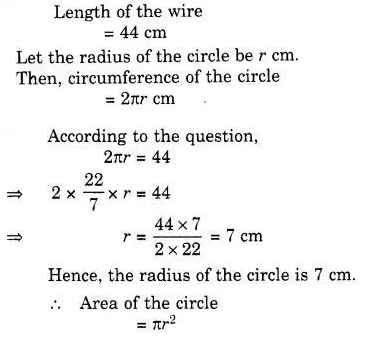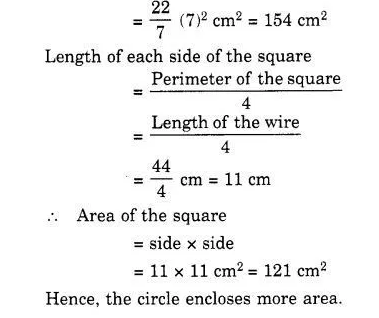# Shazli took a wire of length 44 cm and bent it into the shape of a circle

Shazli took a wire of length 44 cm and bent it into the shape of a circle. Find the radius of that circle. Also find its area. If the same wire is bent into the shape of a square, what will be the length of each of its side? Which figure encloses more area, the circle or the square? ( Take π =$\frac { 22 }{ 7 }$ )